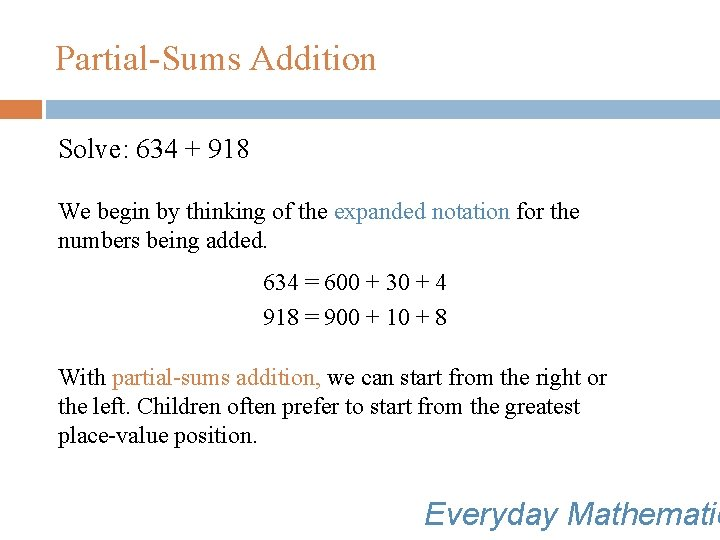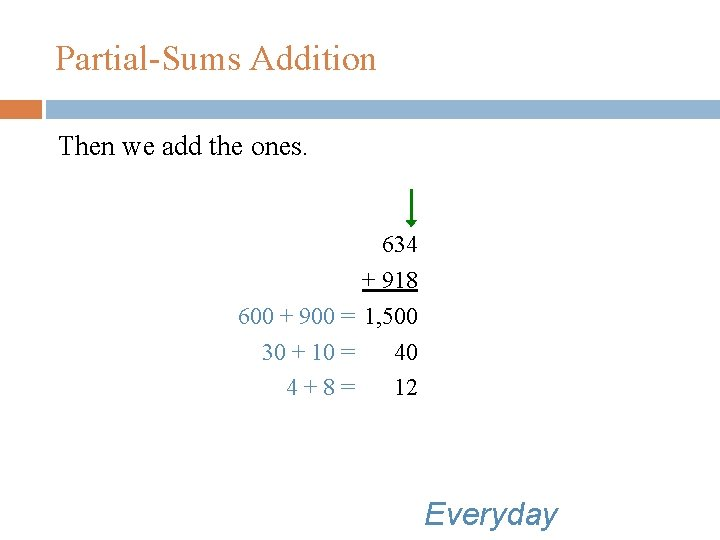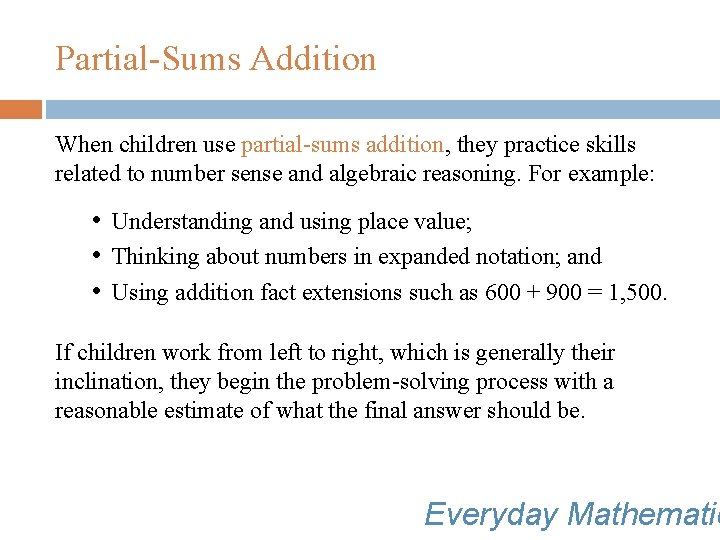• Slides: 8Partial-Sums Addition Partial-sums addition involves: • Understanding place value; • Finding partial sums; and • Adding partial sums to get the final answer. Everyday MathematicPartial-Sums Addition Solve: 634 + 918 We begin by thinking of the expanded notation for the numbers being added. 634 = 600 + 30 + 4 918 = 900 + 10 + 8 With partial-sums addition, we can start from the right or the left. Children often prefer to start from the greatest place-value position. Everyday MathematicPartial-Sums Addition First we add the hundreds. 634 + 918 600 + 900 = 1, 500 The numbers written in blue show our thinking and the numbers written in black show what we write to solve the problem. EverydayPartial-Sums Addition Next we add the tens. 634 + 918 600 + 900 = 1, 500 30 + 10 = 40 EverydayPartial-Sums Addition Then we add the ones. 634 + 918 600 + 900 = 1, 500 30 + 10 = 40 4+8= 12 EverydayPartial-Sums Addition Finally, we add the partial sums to find the answer. 634 + 918 = 1, 552 634+918=1552 634 + 918 1, 500 40 + 12 1, 552 EverydayPartial-Sums Addition When children use partial-sums addition, they practice skills related to number sense and algebraic reasoning. For example: • Understanding and using place value; • Thinking about numbers in expanded notation; and • Using addition fact extensions such as 600 + 900 = 1, 500. If children work from left to right, which is generally their inclination, they begin the problem-solving process with a reasonable estimate of what the final answer should be. Everyday Mathematic